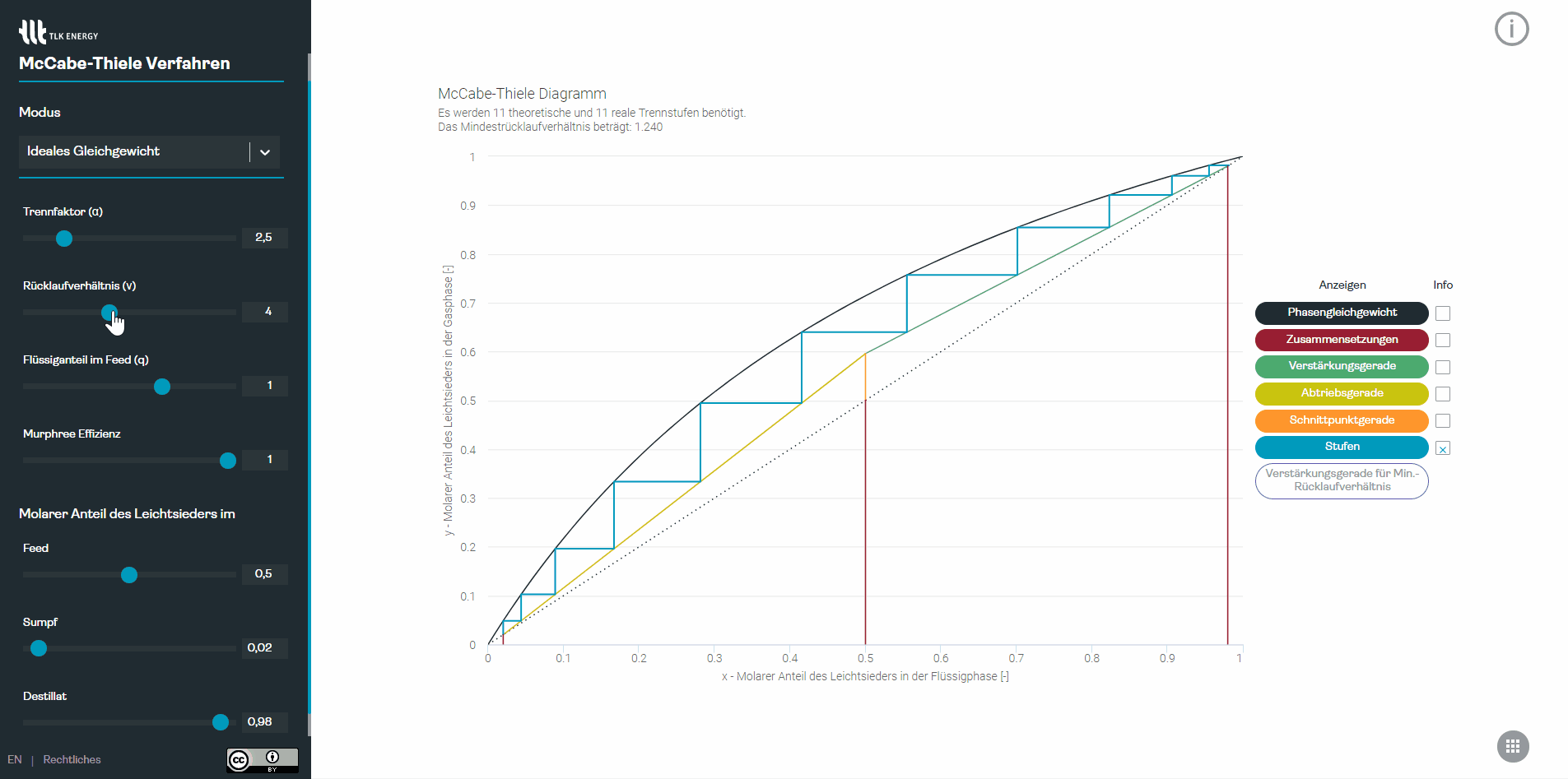July 14, 2021

# Free interactive McCabe-Thiele diagram

Pascal has developed an interactive McCabe-Thiele diagram, which we provide free of charge and without registration. With this diagram from process engineering the theoretical number of separation stages of a column can be determined. How many stages are needed depends on many factors: What is the separation factor of my mixture? What degree of purity is to be achieved? What is the Murphree efficiency and the reflux ratio?
We would appreciate feedback and would be happy to develop the diagram further if it meets interest. 😃